The OpenFOAM FoundationperfectFluid< Specie > Class Template Reference

Simple extension of the perfect gas equation of state to liquids by the addition of a constant density off-set. More...

Inheritance diagram for perfectFluid< Specie >: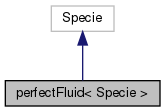[legend]
Collaboration diagram for perfectFluid< Specie >:[legend]

## Public Member Functions

perfectFluid (const Specie &sp, const scalar R, const scalar rho0)
Construct from components. More...

perfectFluid (const dictionary &dict)
Construct from dictionary. More...

perfectFluid (const word &name, const perfectFluid &)
Construct as named copy. More...

autoPtr< perfectFluidclone () const
Construct and return a clone. More...

scalar R () const
Return fluid constant [J/kg/K]. More...

scalar rho (scalar p, scalar T) const
Return density [kg/m^3]. More...

scalar H (const scalar p, const scalar T) const
Return enthalpy contribution [J/kg]. More...

scalar Cp (scalar p, scalar T) const
Return Cp contribution [J/(kg K]. More...

scalar E (const scalar p, const scalar T) const
Return internal energy contribution [J/kg]. More...

scalar Cv (scalar p, scalar T) const
Return Cv contribution [J/(kg K]. More...

scalar Sp (const scalar p, const scalar T) const
Return entropy contribution to the integral of Cp/T [J/kg/K]. More...

scalar Sv (const scalar p, const scalar T) const
Return entropy contribution to the integral of Cv/T [J/kg/K]. More...

scalar psi (scalar p, scalar T) const
Return compressibility [s^2/m^2]. More...

scalar Z (scalar p, scalar T) const
Return compression factor []. More...

scalar CpMCv (scalar p, scalar T) const
Return (Cp - Cv) [J/(kg K]. More...

void write (Ostream &os) const
Write to Ostream. More...

void operator+= (const perfectFluid &)

void operator*= (const scalar)

## Static Public Member Functions

static autoPtr< perfectFluidNew (const dictionary &dict)

static word typeName ()
Return the instantiated type name. More...

## Static Public Attributes

static const bool incompressible = false
Is the equation of state is incompressible i.e. rho != f(p) More...

static const bool isochoric = false
Is the equation of state is isochoric i.e. rho = const. More...

## Friends

perfectFluid operator+ (const perfectFluid &, const perfectFluid &)

perfectFluid operator* (const scalar s, const perfectFluid &)

perfectFluid operator== (const perfectFluid &, const perfectFluid &)

Ostreamoperator (Ostream &, const perfectFluid &)

## Detailed Description

### template<class Specie> class Foam::perfectFluid< Specie >

Simple extension of the perfect gas equation of state to liquids by the addition of a constant density off-set.

Coefficient mixing is very inaccurate and not supported, so this equation of state is not applicable to mixtures.

This equation of state is rather inaccurate and has been superseded by rPolynomial which is much more accurate and supports mixtures.

Source files

Definition at line 54 of file perfectFluid.H.

## ◆ perfectFluid() [1/3]

 perfectFluid ( const Specie & sp, const scalar R, const scalar rho0 )
inline

Construct from components.

Definition at line 32 of file perfectFluidI.H.

References Foam::name().

Here is the call graph for this function: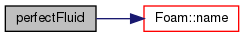## ◆ perfectFluid() [2/3]

 perfectFluid ( const dictionary & dict )

Construct from dictionary.

Definition at line 32 of file perfectFluid.C.

## ◆ perfectFluid() [3/3]

 perfectFluid ( const word & name, const perfectFluid< Specie > & )
inline

Construct as named copy.

## ◆ clone()

 Foam::autoPtr< Foam::perfectFluid< Specie > > clone ( ) const
inline

Construct and return a clone.

Definition at line 61 of file perfectFluidI.H.

References perfectFluid< Specie >::New().

Here is the call graph for this function: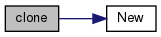## ◆ New()

 Foam::autoPtr< Foam::perfectFluid< Specie > > New ( const dictionary & dict )
inlinestatic

Definition at line 70 of file perfectFluidI.H.

References dict.

Referenced by perfectFluid< Specie >::clone().

Here is the caller graph for this function: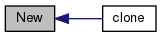## ◆ typeName()

 static word typeName ( )
inlinestatic

Return the instantiated type name.

Definition at line 131 of file perfectFluid.H.

## ◆ R()

 Foam::scalar R ( ) const
inline

Return fluid constant [J/kg/K].

Definition at line 81 of file perfectFluidI.H.

## ◆ rho()

 Foam::scalar rho ( scalar p, scalar T ) const
inline

Return density [kg/m^3].

Definition at line 88 of file perfectFluidI.H.

References R, and T.

## ◆ H()

 Foam::scalar H ( const scalar p, const scalar T ) const
inline

Return enthalpy contribution [J/kg].

Definition at line 95 of file perfectFluidI.H.

References Foam::constant::standard::Pstd, and rho.

## ◆ Cp()

 Foam::scalar Cp ( scalar p, scalar T ) const
inline

Return Cp contribution [J/(kg K].

Definition at line 102 of file perfectFluidI.H.

References Foam::constant::standard::Pstd, R, rho, and Foam::sqr().

Here is the call graph for this function: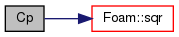## ◆ E()

 Foam::scalar E ( const scalar p, const scalar T ) const
inline

Return internal energy contribution [J/kg].

Definition at line 111 of file perfectFluidI.H.

## ◆ Cv()

 Foam::scalar Cv ( scalar p, scalar T ) const
inline

Return Cv contribution [J/(kg K].

Definition at line 118 of file perfectFluidI.H.

## ◆ Sp()

 Foam::scalar Sp ( const scalar p, const scalar T ) const
inline

Return entropy contribution to the integral of Cp/T [J/kg/K].

Definition at line 125 of file perfectFluidI.H.

References Foam::log(), Foam::constant::standard::Pstd, and R.

Here is the call graph for this function: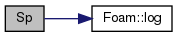## ◆ Sv()

 Foam::scalar Sv ( const scalar p, const scalar T ) const
inline

Return entropy contribution to the integral of Cv/T [J/kg/K].

Definition at line 132 of file perfectFluidI.H.

References NotImplemented.

## ◆ psi()

 Foam::scalar psi ( scalar p, scalar T ) const
inline

Return compressibility [s^2/m^2].

Definition at line 140 of file perfectFluidI.H.

References R, and T.

## ◆ Z()

 Foam::scalar Z ( scalar p, scalar T ) const
inline

Return compression factor [].

Definition at line 147 of file perfectFluidI.H.

## ◆ CpMCv()

 Foam::scalar CpMCv ( scalar p, scalar T ) const
inline

Return (Cp - Cv) [J/(kg K].

Definition at line 154 of file perfectFluidI.H.

References R, rho, and Foam::sqr().

Here is the call graph for this function: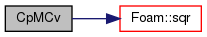## ◆ write()

 void write ( Ostream & os ) const

Write to Ostream.

Definition at line 43 of file perfectFluid.C.

Here is the call graph for this function: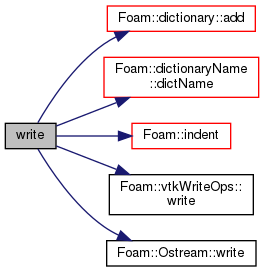## ◆ operator+=()

 void operator+= ( const perfectFluid< Specie > & )
inline

Definition at line 167 of file perfectFluidI.H.

References NotImplemented.

## ◆ operator*=()

 void operator*= ( const scalar s )
inline

Definition at line 176 of file perfectFluidI.H.

References NotImplemented, and s().

Here is the call graph for this function: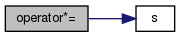## ◆ operator+

 perfectFluid operator+ ( const perfectFluid< Specie > & , const perfectFluid< Specie > & )
friend

## ◆ operator*

 perfectFluid operator* ( const scalar s, const perfectFluid< Specie > & )
friend

## ◆ operator==

 perfectFluid operator== ( const perfectFluid< Specie > & , const perfectFluid< Specie > & )
friend

## ◆ operator

 Ostream& operator ( Ostream & , const perfectFluid< Specie > & )
friend

## ◆ incompressible

 const bool incompressible = false
static

Is the equation of state is incompressible i.e. rho != f(p)

Definition at line 140 of file perfectFluid.H.

## ◆ isochoric

 const bool isochoric = false
static

Is the equation of state is isochoric i.e. rho = const.

Definition at line 143 of file perfectFluid.H.

The documentation for this class was generated from the following files:
• src/thermophysicalModels/specie/equationOfState/perfectFluid/perfectFluid.H
• src/thermophysicalModels/specie/equationOfState/perfectFluid/perfectFluid.C
• src/thermophysicalModels/specie/equationOfState/perfectFluid/perfectFluidI.H